# ADC and DAC: Enhancing Resolution of Conversions Between Analog and Digital Signals

## Tool Used: OptSim

This application note illustrates resolution enhancement of analog-to-digital and digital-to-analog signal conversions in OptSim.

There are three basic signal types in OptSim: logical signal, electrical signal and optical signal. The PRBS data source, for example, outputs a logical signal that a driver model converts into an electrical signal to drive a laser or a modulator. The optical signal at the receiver is converted to an electrical signal by the photodetector. If users need to convert an electrical signal into a logical signal, they can use Analog-to-Digital Converter (ADC) model illustrated below.

Figure 1 shows the schematic layout of the project file that shows the electrical-to-logical signal conversion.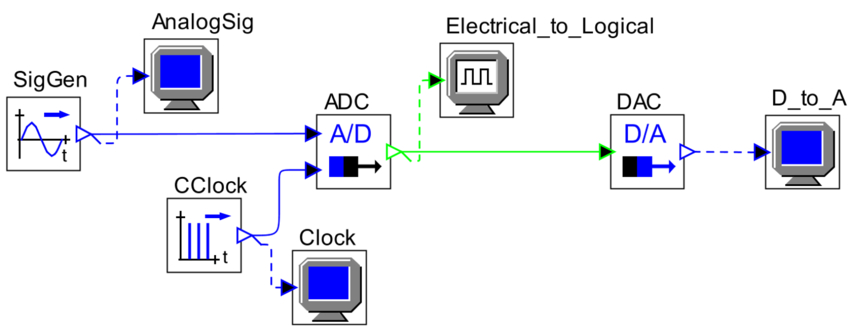Figure 1. Simulation setup schematics for converting an electrical signal to a logical signal

The sine signal generator provides an electrical test signal that we want to convert into digital first, and then back into electrical for verification. The clock drives the A/D converter and sets the sampling rate.

The dynamic range of the A/D converter is set equal to the amplitude of the sine wave. The number of bits used to quantize the electrical signal controls the buffer depth of the logical signals.

The number of bits is set equal to 6 that should be realistic for the current digital signal processors, which one can improve by using a scheme shown in Figure 4.

Figure 2 shows input sine wave (left) and the output signal (right) sampled at 40 samples per period and quantized with 6 bits.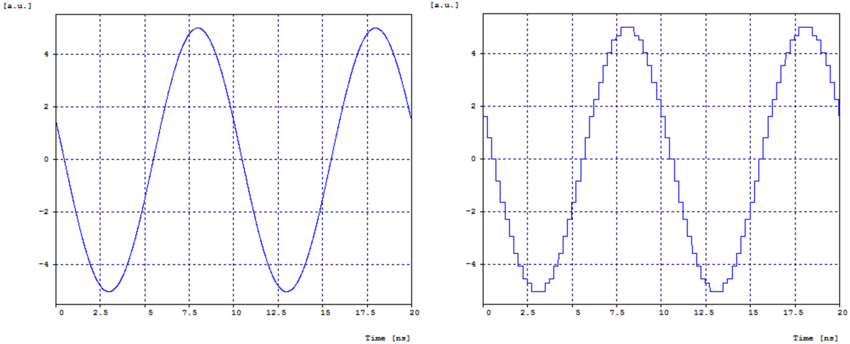Figure 2. Input analog signal (left) and output quantized signal (right)

The representation of the digital values of the A/D and D/A blocks is sign and amplitude. The logical signal probe can be used to display the individual bit values as shown in Figure 3: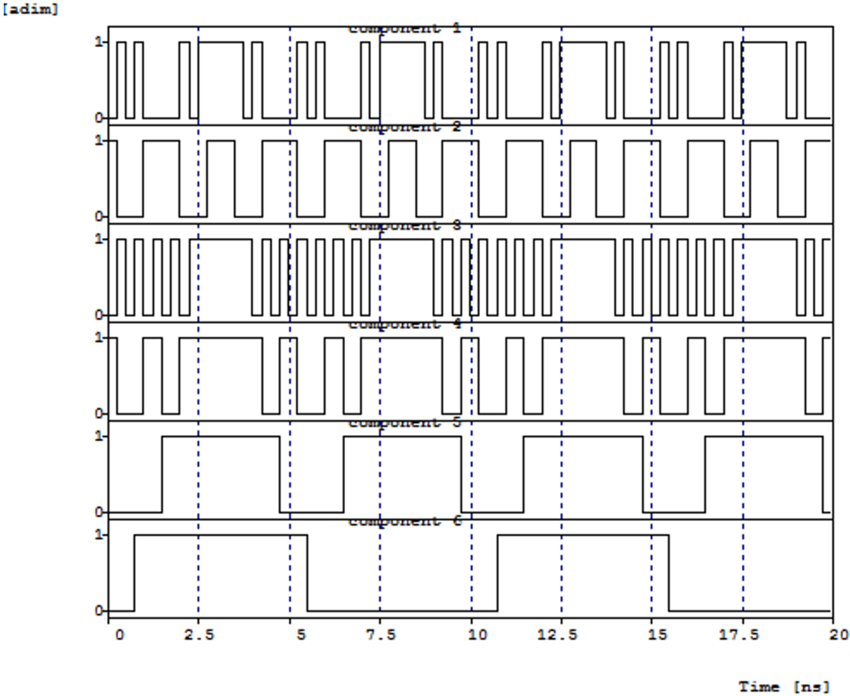Figure 3. Sign and amplitude levels as seen at the logical signal analyzer

As mentioned previously, the blocks A/D and D/A are meant to be used in high-speed DSP units where a typical resolution of 6 to 8 bits is generally enough. However, if needed, one can improve it by using a scheme as shown in Figure 4.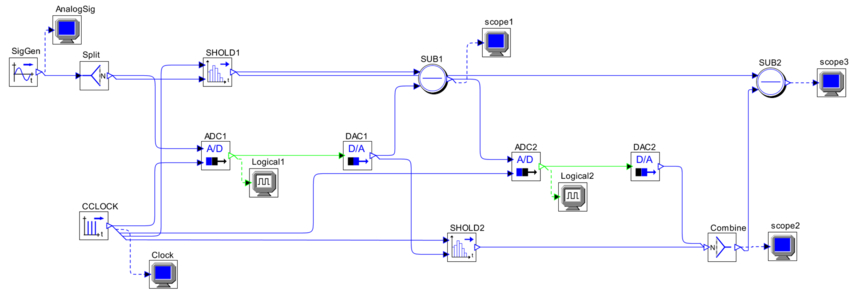Figure 4. Simulation setup schematics for improving resolution of the ADC and DAC models

The method involves cascading stages of ADC and DAC and at each stage convert the quantization error of the previous stage. If the previous stage returns a digital value for the signal, the second stage returns a digital value for the error of the first stage, and so on. To keep the processing synchronous (so that the quantization error is correctly calculated), a couple of sampler and holder blocks are added that wouldn’t otherwise be necessary.

Figure 5 shows quantization error at the output of the first stage (left) and at the second stage (right).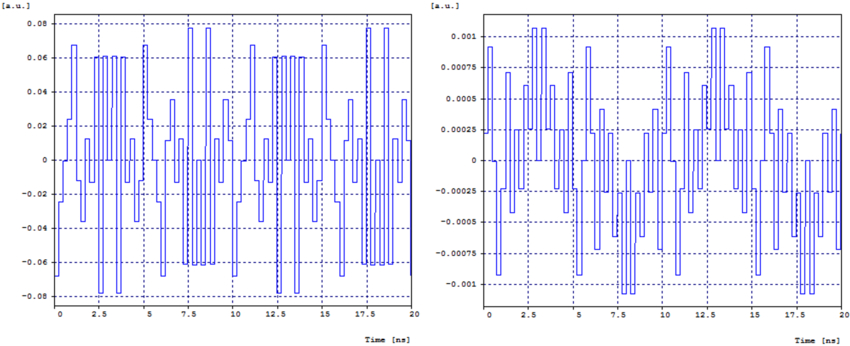Figure 5. Quantization error at the first (left) and second (right) stages

The example uses 6 bits per each A/D and D/A block and an amplitude equal to 5 so the theoretical quantization errors are 5*2-6 = 0.078125 and 5*2-12 = 0.001220703125, which match well with the errors at the output of the first and second stages.

Figure 6 shows bits at the output of the first stage (left) and at the second stage (right).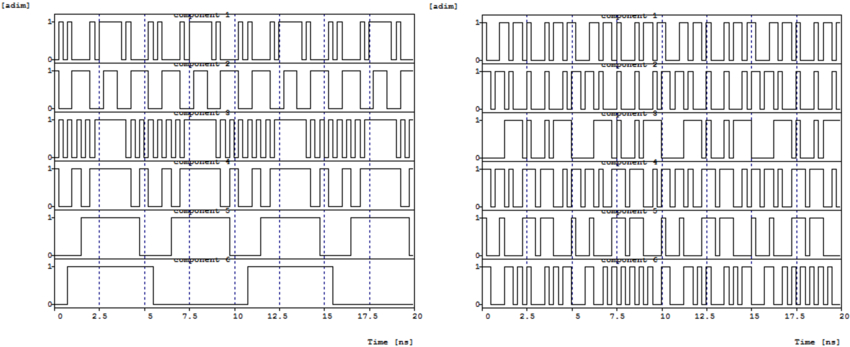Figure 6. Output bits at the first (left) and second (right) stages